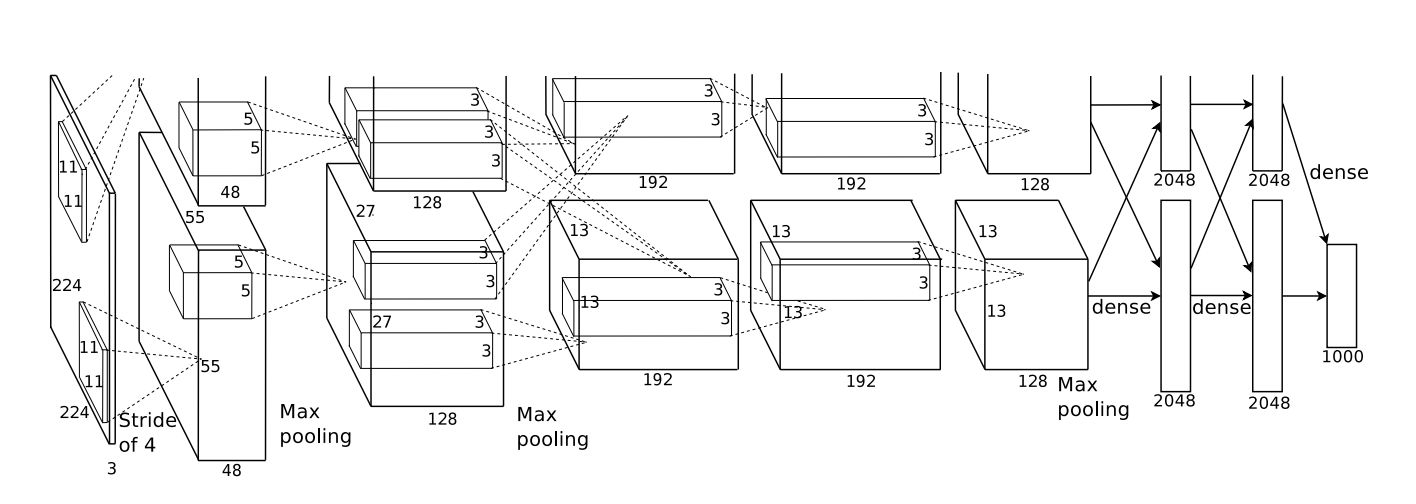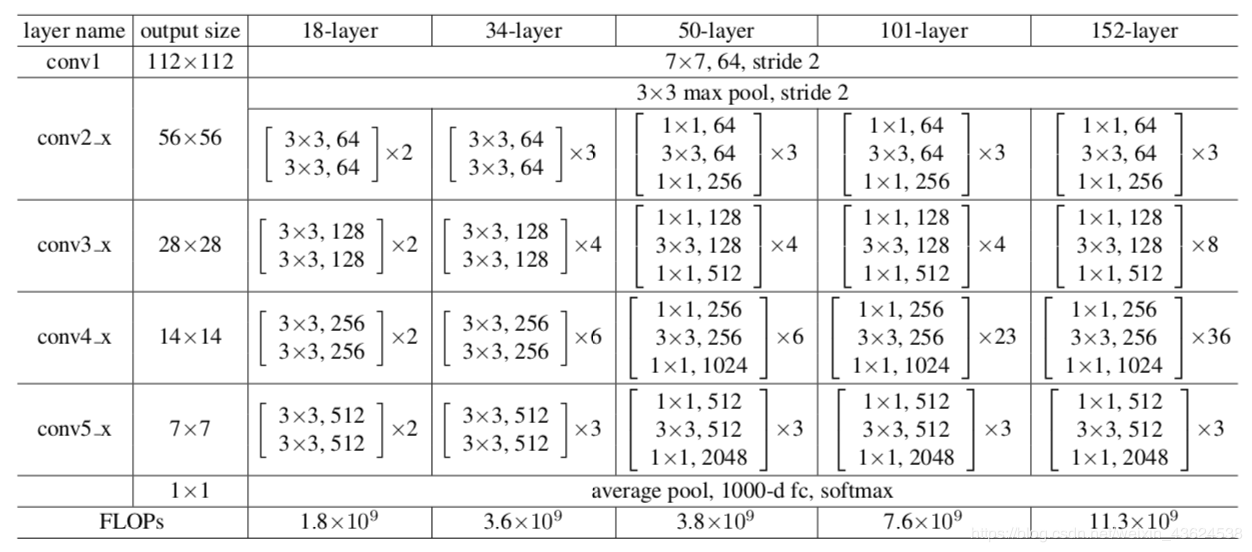# 交叉熵的作用# 激活函数

## Softmax

$softmax(y_{i}) = \frac{e^y_{i}}{\sum_{j=1}^{n}e^y_{i}}$

## Sigmoid

Softmax激活起码要对应N大于等于2的输出结点，那么上述的另一种情况，只有一个结点时处理二分类问题，应该怎么激活？这里就用到了Sigmoid，Sigmoid在早期ANN中常用于去线性化，因为它的特性是将一个数压缩到0-1之间：

$Sigmoid(y) = \frac{1}{1+e^{-y}}$

# 交叉熵原理

## 二值交叉熵

$L(p,t) = -[plog(t)+(1-p)log(1-t)]$

$L_{1}(p,t_{1}) = -log(0.3)=0.522$
$L_{2}(p,t_{2}) = -log(0.6)=0.221$

$L_{1}(p,t_{1}) = -log(1-0.3)=0.154$
$L_{2}(p,t_{2}) = -log(1-0.6)=0.397$

## 交叉熵

$H(P,T) = -\sum_{1}^{C} plog(T)$

$T$为样本的实际输出，其中$T=[softmax(y_{1}),softmax(y_{2}),...,softmax(y_{i=n})]$

$H_{1}(P,T_{1}) =-log(0.3)=0.522$
$H_{2}(P,T_{2}) = -log(0.6)=0.221$

$H_{1}(P,T_{1}) =-(1\times log(0.8)+0\times log(0.1)+0\times log(0.1))=0.096$
$H_{2}(P,T_{2}) = -(1\times log(0.5)+0\times log(0.3)+0\times log(0.2))=0.301$
$T_{1}$要更接近label，所以损失更小。

05-283508

#### 交叉熵损失函数的优点

08-305万+

#### 交叉熵损失函数原理详解09-201万+

#### 为什么用交叉熵做损失函数

06-058645

#### softmax交叉熵损失函数及其求导

11-04547

#### 从理论上理解采用交叉熵作为损失函数的意义

06-121万+

#### 交叉熵损失函数详解

08-143837

#### 神经网络与深度学习笔记（四）为什么用交叉熵代替二次代价函数

05-038058

#### 为什么交叉熵损失函数值越小分类效果越好？

04-0210万+

#### 交叉熵代价函数（作用及公式推导）

07-301万+

#### 关于神经网络中的代价函数——交叉熵的由来©️2020 CSDN 皮肤主题: Age of Ai 设计师: meimeiellie点击重新获取扫码支付1.余额是钱包充值的虚拟货币，按照1:1的比例进行支付金额的抵扣。
2.余额无法直接购买下载，可以购买VIP、C币套餐、付费专栏及课程。余额充值